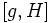# Strongly paranormal implies paranormal

This article gives the statement and possibly, proof, of an implication relation between two subgroup properties. That is, it states that every subgroup satisfying the first subgroup property (i.e., strongly paranormal subgroup) must also satisfy the second subgroup property (i.e., paranormal subgroup)
View all subgroup property implications | View all subgroup property non-implications
Get more facts about strongly paranormal subgroup|Get more facts about paranormal subgroup

## Statement

Any strongly paranormal subgroup of a group is paranormal.

## Definitions used

### Strongly paranormal subgroup

Further information: strongly paranormal subgroup

A subgroup$H \le G$ is termed strongly paranormal if, for any$g \in G$,$[[g,H], H] = [g,H]$. Here,$[g,K]$ is the subgroup generated by all commutators between$g$ and elements of$K$.

### Paranormal subgroup

Further information: paranormal subgroup

A subgroup$H \le G$ is termed paranormal if, for any$g \in G$,$H$ is a contranormal subgroup of$\langle H, H^g \rangle$: in other words, the normal closure of$H$ in$\langle H, H^g \rangle$ is the whole group$\langle H, H^g \rangle$.

## Facts used

1. Product with commutator equals join with conjugate:$H[g,H] = \langle H, [g,H] \rangle = \langle H, H^g \rangle$

## Proof

### Proof idea

The key idea is to show, using the identity, that any normal subgroup of$\langle H, H^g \rangle$ that contains$H$ must also contain$[g,H]$, and hence must be the whole of$\langle H, H^g$.

### Proof details

Given: A group$G$, a subgroup$H$ such that$[[g,H],H] = [g,H]$ for any$g \in G$.

To prove': If$K$ is a normal subgroup of$\langle H, H^g \rangle$ that contains$H$,$K = \langle H, H^g \rangle$.

Proof:

1.$[g,H] \le \langle H, H^g \rangle$: This follows from fact (1).
2.$[[g,H],K] \le K$: Since$[g,H]$ is in$\langle H, H^g \rangle$, we have$[[g,H],K] \le [\langle H, H^g \rangle, K]$. The latter is contained in$K$, since$K$ is normal in$\langle H, H^g \rangle$.
3.$[[g,H],H] \le K$: This follows from the previous step, and the fact that$H \le K$.
4.$[g,H] \le K$: This follows from the previous step, and the fact that$[[g,H],H] = [g,H]$.
5.$K = \langle H, H^g \rangle$: We already have$H \le K$, and the previous fact yields$[g,H] \le K$. Thus, we get$H[g,H] \le K$. By fact (1), this yields$\langle H, H^g \rangle \le K$. Since$K \le \langle H, H^g \rangle$, we get equality.